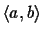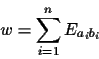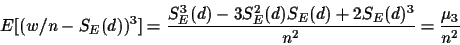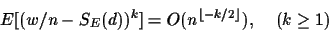Next: How general are these Up: Dayhoff Scores and Evolutionary Previous: Scores are inconsistent to

## How to Make Scores Consistent

As it turns out to be, this is straightforward. For any scoring matrix E for which SE(d) is strictly monotonic we can invert SE(d). Our new score is computed using the inverse, i.e.

 d* = SE-1(w/n) (6)

where w is the actual score obtained from a pair of aligned sequences.(7)

For n sufficiently large, w/n converges to its expected value SE(d) and d* converges to d. Since SE(d) is a sum of exponentials in d, it is generally not possible to invert it algebraically. The computation of the inverse will be done numerically. Letbe the kth moment of the scoring matrix E. The central moments of w/n are:

E[w/n] = SE(d)(8)(9)(10)Next: How general are these Up: Dayhoff Scores and Evolutionary Previous: Scores are inconsistent to
Chantal Korostensky
1999-07-14